# Logic Gates : Definitions, Types and Truth Table

Sharing is Caring :)-

Today we will discuss about logic gates, its types and definitions, and uses. Logic gates are widely used in electronic industries and robotics. Basically, the elements of (logic gates) are build of block which is performed by digital circuits. Boolean algebra function may be performed by digital circuits. It is an electronic circuit which is design base on 0 or 1. All of the Boolean function will be operated on value depends on 0 and 1. The function will give more input to the circuit but one output will become out from a circuit. Relation between input and output is based on a certain logical gate.

The circuit element which performs all this Boolean function is called logic gates. They are represented by some special symbols. This symbol will represent which type of function is performed by a logic gate.

# Types of Logic Gates:

The most common types of logic gate is mention below –

1. Or-gate
2. And-gate
3. Not-gate
4. Nand-gate
5. Nor-gate
6. E-nor (exclusive-nor gate)
7. E-or (exclusive-or gate)

Not and Nand gates are called universal gates.

All combinational logic gate is constructed with and, or, and not.[example-Nand (not+and) and nor (not+or) gate].

Also Read : Types of Servomotor

Also Read : What is Mechatronics?

### 1. Or-gate

Or gate has two and more inputs terminal but we get only one output terminal. In a or gate two and more than inputs signal are high then output signal becomes high. If two and more than inputs signal are low then an output signal becomes low.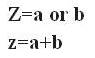Inputs

Output(z)

A

B

0

0 0

0

1

1

1 0

1

1

1

1

### 2. And-gate

And gate has two and more inputs terminal but we get only one output terminal. In a or gate two and more than inputs signal are high then output signal becomes high. This gate is only provided high input.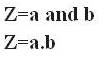Inputs

Output(z)
A

B

0

0 0

0

1 0
1 0

0

1 1

1

### 3. Not-gate:

In not gate only one input and one output. A not-gate is known as an inverter gate because the input will be just the opposite of an output example when input is 0 then the output will come out 1, input is 1 then the output will be 0. So is called an inverter gate.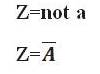Input Output
0 1
1 0

### 4. Nand-gate:

Nand gate is a combinational logic gate is constructed with not gate and and gate (not+and). Nand gate has two and more inputs terminal but we get only one output terminal. In gate, not gate works as an inverter. The nand gate all inputs are high and output will be low. It acts as a negative or gate.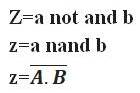Inputs

Output(z)

A

B

0

0 1
0 1

1

1

0 1
1 1

0

### 5. Nor-gate:

Nor-gate is a combinational logic gate is constructed with not gate and and gate (not+or). Nor-gate has two and more inputs terminal but we get only one output terminal, all input must be low to provide high output. It acts as a negative and gate.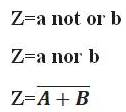Inputs

Output(z)

A

B

0

0 1

0

1 0
1 0

0

1 1

0

### 6. Ex-nor (exclusive-nor gate):

Ex-nor gate is a coincidence gate. It has two and more input terminals and one output terminal. It provides when two high inputs are same output produce high, two inputs are low are same output produce low.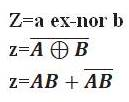Inputs

Output(z)

A

B

0

0 1
0 1

0

1

0 0
1 1

1

### 7. Ex-or (exclusive-or gate):

ex-or (exclusive-or gate) gate is an anti-coincidence gate. It is an inequality detector gate. It has two and more input terminals and one output terminal. It provides when two high inputs are different output produce high, two inputs are low are different output produce low.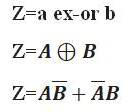Inputs

Output(z)

A

B

0

0 0
0 1

1

1

0 1
1 1

0

### Representation logic gates with ic and symbols: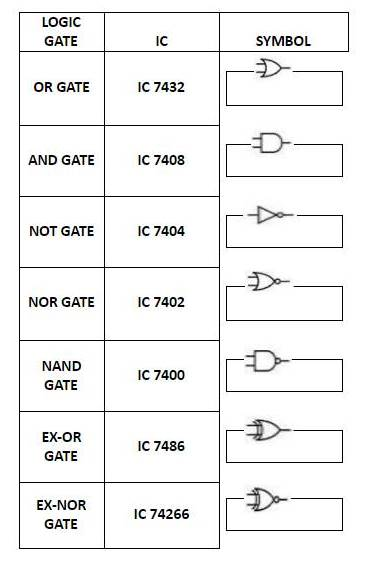This is brief introduction about logic gates. These gates are widely used in mechatronic and robotics industries and all smart devices work on its logics.

Sharing is Caring :)-